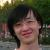为什么 C++ 成员函数指针是 16 字节宽的已翻译 100%

oschina 投递于 2014/12/02 07:23 (共 4 段, 翻译完成于 12-02)

参与翻译 (2人) : 泥牛, pseudo

Pushes and pops on the stack are always in 8-byte strides, and pointers are 8 bytes wide.

#include <iostream>

int main() {
std::cout <<
"sizeof(int*)      == " << sizeof(int*) << "\n"
"sizeof(double*)   == " << sizeof(double*) << "\n"
"sizeof(void(*)()) == " << sizeof(void(*)()) << std::endl;
}

\$ uname -i
x86_64
\$ g++ -Wall ./example.cc
\$ ./a.out
sizeof(int*)      == 8
sizeof(double*)   == 8
sizeof(void(*)()) == 8

#include <iostream>

struct Foo {
void bar() const { }
};

int main() {
std::cout << sizeof(&Foo::bar) << std::endl;
}

ptr：

struct A {
void foo() const { }
};

struct B {
void bar() const { }
};

struct C : A, B
{ };

#include <iostream>

struct A {
void foo() const {
std::cout << "A's this: " << this << std::endl;
}
};

struct B {
void bar() const {
std::cout << "B's this: " << this << std::endl;
}
};

struct C : A, B
{ };
\$ g++ -Wall -o test ./test.cc && ./test
A's this: 0x7fff57ddfb48
B's this: 0x7fff57ddfb68

void call_by_ptr(const C &obj, void (C::*mem_func)() const) {
(obj.*mem_func)();
}

#include <iostream>

struct A {
void foo() const {
std::cout << "A's this:\t" << this << std::endl;
}
};

struct B {
void bar() const {
std::cout << "B's this:\t" << this << std::endl;
}
};

struct C : A, B
{ };

void call_by_ptr(const C &obj, void (C::*mem_func)() const)
{
void *data;
std::memcpy(data, &mem_func, sizeof(mem_func));
std::cout << "------------------------------\n"
"Object ptr:\t" << &obj <<
"\nFunction ptr:\t" << data <<
"\nPointer adj:\t" << data << std::endl;
(obj.*mem_func)();
}

int main()
{
C obj;
call_by_ptr(obj, &C::foo);
call_by_ptr(obj, &C::bar);
}

------------------------------
Object ptr:    0x7fff535dfb28
Function ptr:  0x10c620cac
A's this:    0x7fff535dfb28
------------------------------
Object ptr:    0x7fff535dfb28
Function ptr:  0x10c620cfe
B's this:    0x7fff535dfb48

评论(19)

S
void foo()
{
cout<<"hello world"<<endl;
}
class A
{
public:
void bar()
{
cout<<"A"<<endl;
}
};

class B
{
public:
void barB()
{
cout<<"B"<<endl;
}
};

class C:public A,public B
{
};

int main()
{
typedef void (*f)() ;
typedef void (C::*mem_f)();
f f_ins = &foo;

A* a = new A;
//mem_f f_ins_member = &a->bar;
cout<<sizeof(f_ins)<<endl;
cout<<sizeof(&(C::bar))<<endl;
system("pause");
return 0;
}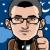引用来自“娱乐你我”的评论

"一个成员函数指针是 16 位的，因为除了需要 8 位字节来存储函数的地址外" 应该是“16字节” 和 “8字节” 不是“位”。
#include <iostream>

struct Foo {
void bar() const { }
};

int main() {
std::cout << sizeof(&Foo::bar) << std::endl;
} 这个x86下，vc编译器，试过显示4字节。不过在多重继承以后再去打印成员函数确实是8字节（就后面那个例子打印mem_func长度）。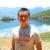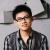引用来自“夫复何求”的评论

sizeof(int*) == 4
sizeof(double*) == 4
sizeof(void(*)()) == 4
[Finished in 0.1s]
me too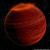Delphi也是这样的，函数指针同样隐藏了一个this（self）指针，要不然它无法指向正确的数据，我为这个问题曾经困惑了很久，后来就明白了。今天看到你的文章就更清楚了。不过不光x64这样，x32也是这样，希望楼主能够补充一个x32的例子，毕竟更普遍。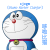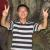C++中的坑比较多,实际开发中一不小心就会陷入.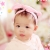引用来自“Raymin”的评论

C++ 的复杂性由此可见一斑！